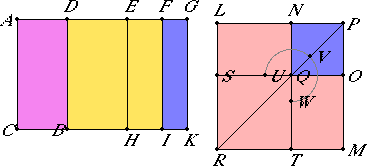# Proposition 95

If an area is contained by a rational straight line and a fifth apotome, then the side of the area is a straight line which produces with a rational area a medial whole.

Let the area AB be contained by the rational straight line AC and the fifth apotome AD.

I say that the side of the area AB is a straight line which produces with a rational area a medial whole.X.Def.III.5

Let DG be the annex to AD. Then AG and GD are rational straight lines commensurable in square only, the annex GD is commensurable in length with the rational straight line AC set out, and the square on the whole AG is greater than the square on the annex DG by the square on a straight line incommensurable with AG.

X.18

Therefore, if there is applied to AG a parallelogram equal to the fourth part of the square on DG and deficient by a square figure, then it divides it into in commensurable parts.

Bisect DG at the point E, apply to AG a parallelogram equal to the square on EG and deficient by a square figure, and let it be the rectangle AF by FG. Then AF is incommensurable in length with FG.

X.21

Now, since AG is incommensurable in length with CA, and both are rational, therefore AK is medial.

X.19

Again, since DG is rational and commensurable in length with AC, therefore DK is rational.

VI.26

Now construct the square LM equal to AI, and subtract the square NO, equal to FK and about the same angle, the angle LPM. Then the squares LM and NO are about the same diameter. Let PR be their diameter, and draw the figure.

Similarly then we can prove that LN is the side of the area AB.

I say that LN is the straight line which produces with a rational area a medial whole.

Since AK was proved medial and equals the sum of the squares on LP and PN, therefore the sum of the squares on LP and PN is medial.

Again, since DK is rational and equals twice the rectangle LP by PN, therefore the latter is itself also rational.

And, since AI is incommensurable with FK, therefore the square on LP is also incommensurable with the square on PN. Therefore LP and PN are straight lines incommensurable in square which make the sum of the squares on them medial but twice the rectangle contained by them rational.

X.77

Therefore the remainder LN is the irrational straight line called that which produces with a rational area a medial whole, and it is the side of the area AB.

Therefore the side of the area AB is a straight line which produces with a rational area a medial whole.

Therefore, if an area is contained by a rational straight line and a fifth apotome, then the side of the area is a straight line which produces with a rational area a medial whole.

Q.E.D.

## Guide

This proposition is used in X.109.